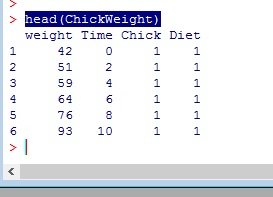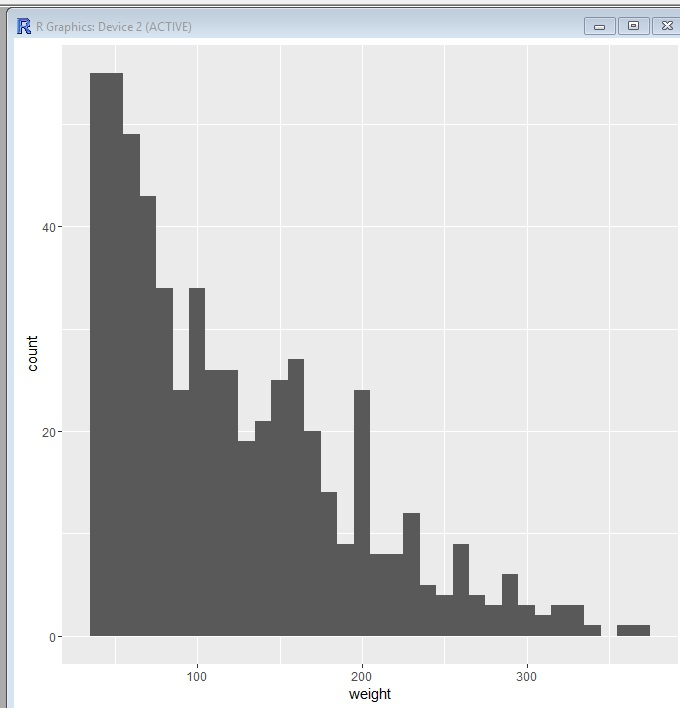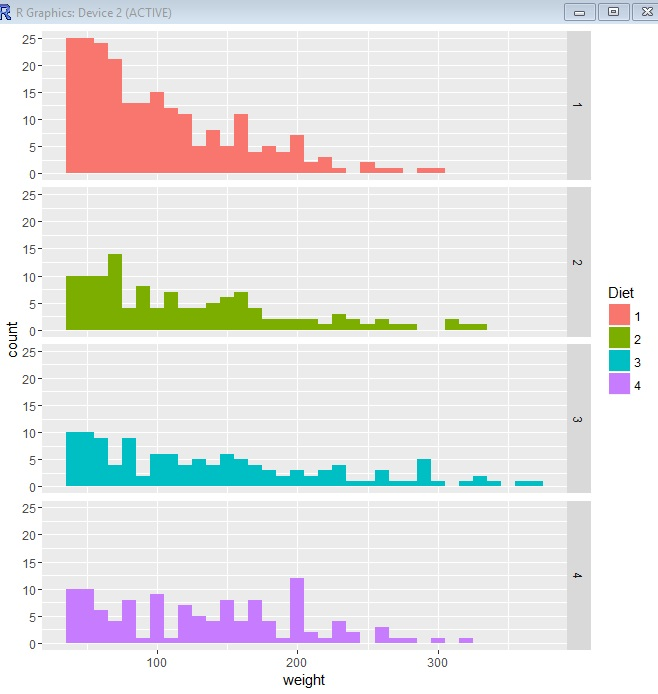# R: ggplot using facets

In this example I am going to teach you to use facets to add another level to your ggplot data visualizations. In this example we will be working with the ChickWeight data set from R Datasets

First, let’s check out the data by running head()

`head(ChickWeight)`We have 4 columns in our data, weight, Time, Chick, and Diet.

Now, let’s build our ggplot model. Below, we are setting our model to a variable “p1”. We then set ChickWeight as our data set and the weight column to our x axis. Remember, nothing will happen when running the line below.

`p1 <- ggplot(data=ChickWeight, aes(x=weight))`

Now lets graph our plot as a histogram – we will set our binwidth to 10 – if any of this confusing you, go back and check out my earlier lessons on ggplot and ggplot histograms

`p1 + geom_histogram(binwidth = 10)`Let’s now add some color. I am going to set our “fill” to be Diet

`p1 + geom_histogram(binwidth = 10, aes(fill=Diet))`Now, using facets, I am going to take it one step further and separate this into 4 histograms, one for each diet.

` p1 + geom_histogram(binwidth = 10, aes(fill=Diet)) + facet_grid(Diet~.)`## The Code

```#-- Look at data

#-- build ggplot model
p1 <- ggplot(data=ChickWeight, aes(x=weight))

#--plot ggplot as histogram
p1 + geom_histogram(binwidth = 10)

#-- set fill color to Diet
p1 + geom_histogram(binwidth = 10, aes(fill=Diet))

#-- create four histograms - one for each Diet
p1 + geom_histogram(binwidth = 10, aes(fill=Diet)) + facet_grid(Diet~.)```